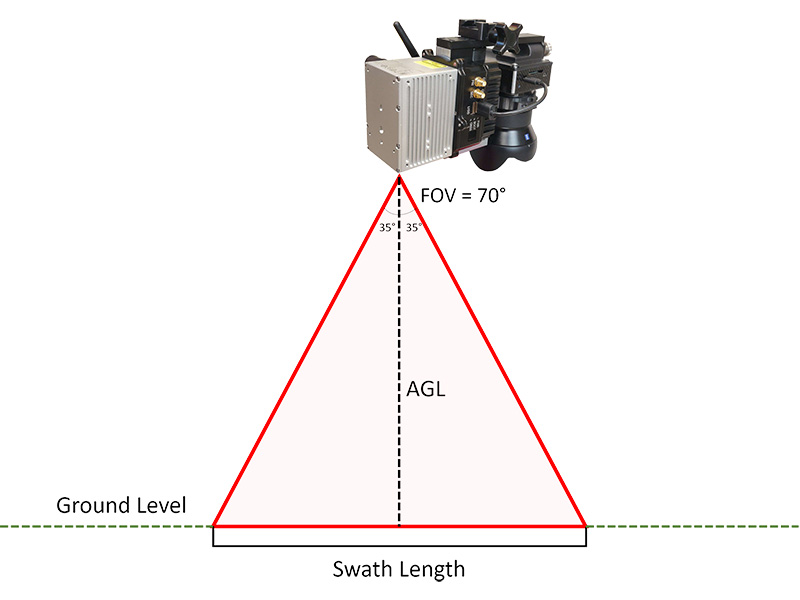## How Can I Estimate the LiDAR Point Density for My Flight?

Purpose: To get a better understanding of the necessary distance between flight passes for your project.
Last Updated: May 2021

Firstly, it is important to note that the point density of a project is dependent on the following listed factors.

• LiDAR scanner field of view
• Flight AGL (Above Ground Level)
• Flight Speed
• LiDAR Scan Rate

First, to understand the area you are covering you must find the swath length. Below is an illustration of what a swath would look like in the case of a payload with Livox Avia.*Drawing Not to Scale

Where:

Swath Length = AGL (m) *(2*tan(FOV/2))

So in this example let AGL = 100 meters. Our swath length would be as follows:

Swath Length = 100*(2*tan(35°))
Swath Length ≈ 140 meters

Once our swath length is determined, we can find the point density of the scan using the following formula:

Point Density = LiDAR Scan Rate (pts/s)/(Swath Length (m) * Flight Speed (m/s))

So for our example let’s say we flew at 5 m/s, then our point density would be as follows:

Point Density = 240,000/(140*5) ≈ 343 pts/m2
Point Density ≈ 343 pts/m2